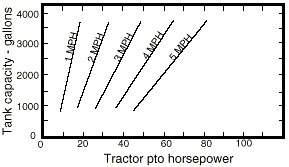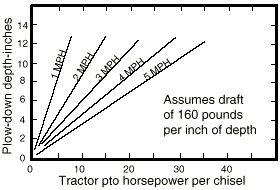# Operating Considerations for Biosolids Equipment

The land area required for biosolids depends upon the amount and characteristics of biosolids produced, and the crops, soils characteristics and agronomic practices used. The amount of land that must be covered influences decisions on types and sizes of equipment needed to manage biosolids. The following table is an estimate of land area requirements for various size municipalities.

Table 1
Estimate1 of area required for land application of biosolids for different size municipalities.

PopulationArea,2 acres
3,00030
5,00050
7,00070
10,000100
20,000200
50,000500
##### 1These values are estimates only. Actual land area requirements must be based on the amount and characteristics of biosolids produced, and the crops, soils, and agronomic practices utilized.2Assumes 100 dry tons biosolids per year per 5,000 people. This is typical for treatment plants with extended aeration. Activated sludge plants, or trickling filters may yield up to 40 percent more biosolids than plants with extended aeration. Biosolids application rate assumed to be 2 dry tons per acre per year.

Biosolids application equipment should be selected and managed with the above acreages in mind.

Many operational requirements or considerations must be addressed in the land application of biosolids. A primary objective in biosolids application is to apply a target amount of nutrients to the land as precisely as possible. This requires proper coordination of pumping rate, or applicator discharge rate and applicator travel speed with the equipment discussed above. Other applicable factors include nutrient concentration in the biosolids and the desired nutrient application rate (pounds per acre). The following formulas can be used to calculate appropriate parameters for the application of liquid biosolids. For similar calculations involving cake biosolids (spread with mechanical spreaders) see MU publications WQ213, Measuring Manure Spreader Uniformity.

Area covered, travel speed, applicator width:

#### Equation 1

A = 0.1212 x S x W

##### A = area covered, acres per hourS = applicator travel speed, miles per hourW = effective applicator width, feet

Nutrient concentration, application rate, discharge rate:

#### Equation 2

S = (C x D x 0.00415)/ R x W

##### C = nutrient concentration in biosolids, wet basis or "as is," ppmD = applicator discharge rate, gallons per minuteR = nutrient application rate, pounds per acre

The following conversion formula may be useful in obtaining wet basis nutrient concentration:

nutrient concentration, wet basis = nutrient concentration, dry basis x percent dry matter/100

Travel speed, gallons spread per acre:

#### Equation 3

S = (D x 495)/G x W

##### G = gallons spread per acre

Example 1
Certain biosolids have a nitrogen concentration (wet basis) of 1,620 ppm and a dry matter content of 3 percent. It is desired to land-apply these biosolids such that the nitrogen application rate is 180 pounds per acre. The applicator to be used has 4 injector knives on 2.5-foot spacing (effective applicator width = 4 x 2.5 = 10 feet), and is capable of discharging biosolids at a rate of 450 gpm.

What travel speed should the operator use to obtain the desired nitrogen application rate, how many gallons will be spread per acre, and at what rate (acres per hour) will the area be covered?

Travel speed is calculated from Equation 2.

S = (1,620 x 450 x 0.00415)/ 180 x 10 = 1.7 miles per hour

Gallons spread per acre is calculated from Equation 3.

G = (450 x 495)/1.7 x 10 = 13,100 gallons per acre

Acres covered per hour is calculated from Equation 1.

A = 0.1212 x 1.7 x 10 = 2.06 acres per hour

Example 2
What travel speed should be used if it is desired to apply two dry tons per acre biosolids?

In this case, the nutrient is dry tons, and the application rate is 4,000 pounds per acre. The wet basis nutrient concentration is 3 percent, or 30,000 ppm. Equation 2 is then calculated as follows.

S = (30,000 x 450 x 0.00415)/4,000 x 10 = 1.4 miles per hour

The above equations can be manipulated algebraically to solve for any of the desired parameters.

Another consideration in off-site application may be power requirements for applicators. This is especially true in the case of farm tractors that may be used to pull tank wagons that inject biosolids into the ground. Figures 1 and 2 show approximate power requirements for pulling loaded tank wagons and injector knives through soil. These figures in equation form are as follows.Figure 1
Approximate tractor size (PTO Horsepower) needed to pull a tank wagon of a given capacity through a plowed field at speeds of 1 to 5 miles per hour.Figure 2
Approximate tractor size (PTO Horsepower) needed to inject biosolids with chisels at speeds shown, in heavy soils and sod.

#### Equation 4

HPT = (0.002129 x S + 0.001265)V + (7.9755 x S) - 3.7525

#### Equation 5

HPI = 0.566 x S x D x N

##### S = speed traveled, miles per hourV = tankwagon volume, gallonsD = depth of chisels, inchesN = number of chisels

Example 3
A 4,000-gallon tank wagon with a 5-chisel injection unit is available to land-apply biosolids. If the tankwagon is pulled at 4 miles per hour, and the biosolids are injected to a depth of 10 inches, what approximate tractor horsepower will be required?

Power required to pull the tank wagon:

HPT = (0.002129 x 4 + 0.001265) 4,000 + (7.9755 x 4) - 3.7525 = 67 horsepower

Power required to pull the injector unit:

HPI = 0.566 x 4 x 10 x 5 = 113 horsepower

Total power requirement:

67 + 113 = 180 horsepower

Another consideration in the off-site application of biosolids is the effect of travel time (distance of the site from the treatment plant) on the field efficiency of the applicator. As travel time for the tanker increases, applicator downtime in the field will increase because there will be more waiting time for the tanker. Increasing the number of tankers and the volume of the tankers relative to the applicator will reduce this downtime. The following equation provides an estimate of the percent downtime (time the applicator is waiting, neither loading or applying) for the applicator as a function of several variables.

PDT = ((TV/AV) TAL + (TV/AV - 1) TAU + RT - N (TV/AV) (TAL + TAU)) / ((TV/AV) TAL + (TV/AV - 1) TAU + RT) x 100

##### PDT = percent downtime for applicator in fieldTV = tanker volume, gallonsAV = applicator volume, gallonsTAL = time to load applicator from tanker, minutesTAU = time to unload, or apply from applicator, minutesRT = round trip time for tanker (site to treatment plant, load and back to site), minutesN = number of tankers

Example 4
A 3,000 gallon applicator is to be supplied by a 3,000 gallon tanker in an off-site biosolids operation. Average time to load the applicator from the tanker is 12 minutes. Average time for the applicator to apply the 3,000 gallon load is 10 minutes. One-way travel time for the tanker is 20 minutes, and loading time at the treatment plant averages 10 minutes, for a round-trip total of 50 minutes.

What percent applicator downtime could be expected in this operation? What would be the effect of using two 3,000 gallon tankers? What would be the effect of using one 6,000 gallon tanker?

PDT = ((3,000 ÷ 3,000) 12 + (3,000 ÷ 3,000 - 1) 10 + 50 - 1 (3,000 ÷ 3,000) (12+10)) / ((3,000 ÷ 3,000) 12 + (3,000 ÷ 3,000 - 1) 10 + 50) x 100 = 64.5 percent

If two 3,000 gallon tankers were used, PDT is calculated as follows.

PDT = ((3,000 / 3,000) 12 + (3,000 / 3,000 - 1) 10 + 50 - 2 (3,000 / 3,000) (12 + 10)) / ((3,000 / 3,000) 12 + (3,000 / 3,000 - 1) 10 + 500) x 100 = 29 percent

If one 6,000 gallon tanker were used, PDT is calculated as follows.

PDT = ((6,000 / 3,000) 12 + (6,000 / 3,000 - 1) 10 + 50 - 1 (6,000 / 3,000) (12+10) ) / ((6,000 /; 3,000) 12 + (6,000 / 3,000 - 1) 10 + 50) x 100 = 47.6 percent

The effect of faster loading and unloading times, or reducing round trip time by pumping part way to the site, can be evaluated by adjusting the appropriate inputs. A negative value for PDT indicates no waiting time for the applicator (no downtime).